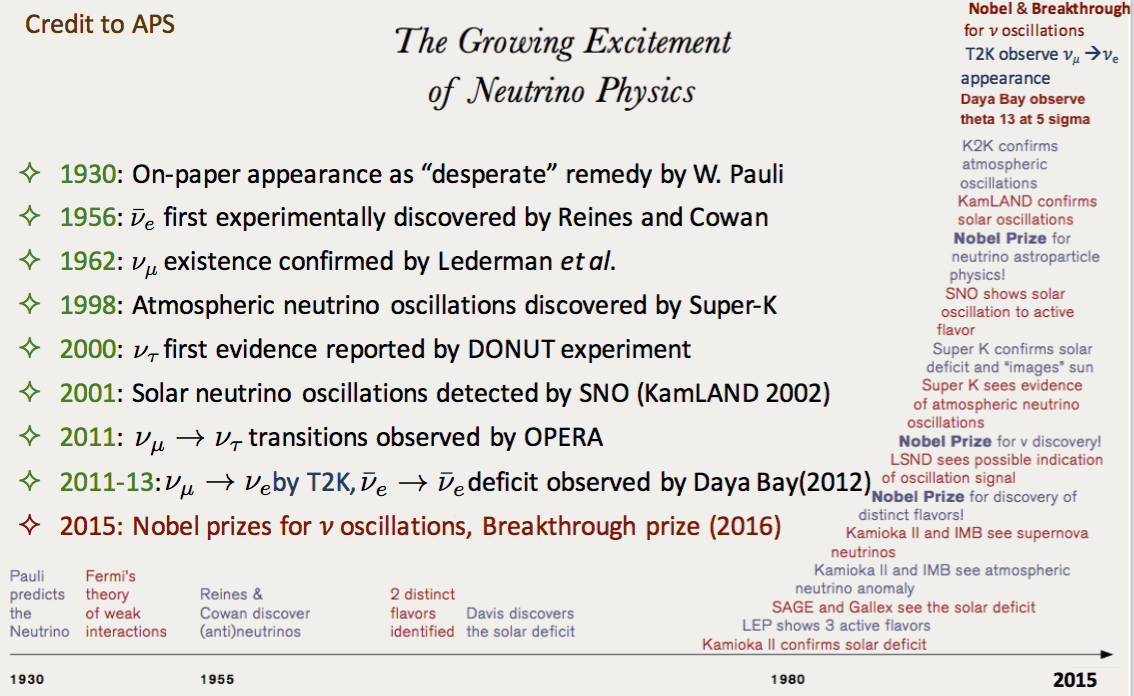English | Tiếng Việt

Neutrinos Physics

Since the first on-paper appearance as a desperate remedy proposed by W. Pauli for resolving the beta decay issue in 1930, neutrino is still surprising physicists and is a main stream to explore the physics beyond the Standard Model (SM). The Nobel prize in 2015 and the Breakthrough prize in 2016 are the valuable words for the vital role of neutrino in the modern physics. After seven decades, the picture of neutrino physics is fairly shaped. However some mysteries are still hidden at some corners.Brief history of neutrino physics, taken from S. Cao’s slide at PASCOS 2016

Nobel Prizes for neutrino research
1. In 1988, “for the neutrino beam method and the demonstration of the doublet structure of the leptons through the discovery of the muon neutrino”, Leon M. Lederman, Melvin Schwartz and  Jack Stecinberger shared the first Nobel Prize relating to neutrino research
2. In 1995, Frederick Reines was received the Nobel Prize for detecting the first neutrino in 1956
3. In 2002, Raymond Davis Jr. and Masotoshi Koshiba shared the Nobel Prize for detecting cosmic neutrinos
4. In 2015, Takaaki Kajita and Arthur B. Mc Donald have received the Nobel Prize for discovery of neutrino oscillation, showing that neutrinos have mass.

What do we know about neutrino?

Neutrinos are one of the elementary particles which make up the Universe. They are almost similar to the more familiar electron with one crucial exception: neutrinos do not carry electri charge. That means they are not affected by the electromagnetic forces. If gravity doesn’t take into account because of neutrino’s small mass, neutrinos interact only via the weak force.
There is three types of neutrino, or sometime called “three flavors of neutrinos”: electron neutrino 𝜈e, muon neutrino 𝜈μ, and tau neutrino 𝜈𝜏. Each flavor of neutrino is related to a charged particle (corresponding to neutrino its name). For example, electron neutrino 𝜈e is associated with the electron.

Neutrinos have mass, although their mass is much smaller than electron (< 4/106 electron mass). This fact is indeed beyond the description of Standard Model of elementary particle (neutrino is predicted to be massless in this model). The evidence of neutrino massiveness comes from the now well-understood neutrino oscillation phenomenon in which neutrinos change their flavor when traveling. This quantum mechanical phenomenon is widely accepted as the result of mixing between mass eigenstates and flavor eigenstates (see more in Neutrino Oscillation). The element of mixing matrix which are parameterized by three mixing angles, two mass-squared difference and one irreducible phase 𝛿CP, are the target for the experimental measurement. Bellow graph summarizes what we do know for this mixing matrix. The phase 𝛿CP is very interesting since it relates to CP violation in neutrino sector and might connect to the leptogenesis as introduced in CP violation search

What do we not known about neutrino?

Following is some opening question we would like to address in neutrino physics.
• CP violation in neutrino sector? How does it relate to the leptongenesis?
• Can be measured by comparing neutrino and anti-neutrino oscillations
• What is the value of 𝛿CP?
• How do neutrinos get mass?
• Higgs mechanism? Seesaw mechanism
• Is neutrino Majorana particles?
• Neutrino mass hierarchy?
• If inverted, so why neutrino is different from other elementary particles?
• Is the mixing angle 𝜃23 maximum?
• If so, what’s the underlying symmetry?
• Is sterile neutrinos existed?
• If so, how a new model can describe these particles?
• Is the mixing matrix unitary?
• If not, what does it mean?What we know and what we don’t know, from S. Cao’s slide at PASCOS 2016